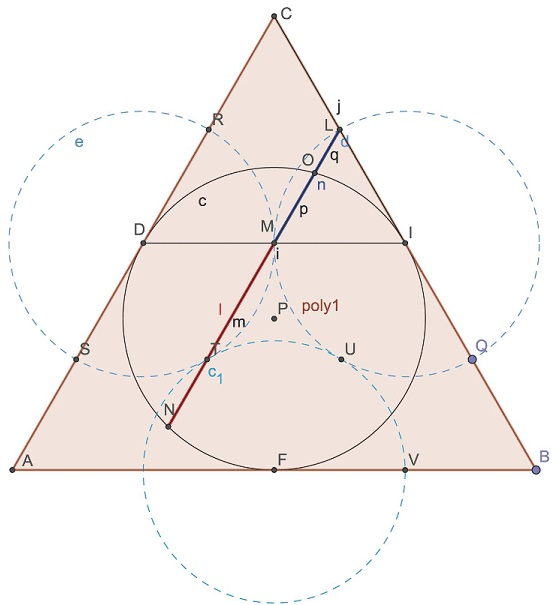# Golden Ratio In Three Tangent Circles

### Tran Quang Hung's variant

Given three pairwise tangent circles $(A),\;$ $(B),\;$ and $(C),\;$ let $F,\;$ $D,\;$ $E\;$ be the points of tangency. Line $DF\;$ intersects circles $(B),\;$ $(C),\;$ and $(ABC)\;$ successively in points $G,\;$ $H,\;$ $F,\;$ $D,\;$ $I,\;$ $J.$Then $\displaystyle\frac{FH}{GH}=\frac{DF}{FH}=\frac{DH}{DJ}=\varphi,\;$ the Golden Ratio.

Assume $L\;$ is the shared center of $(ABC)\;$ and $(DEF)\;$ and that $DE=1.\;$ Also let $K\;$ be the midpoint of $DF.\;$Then $AB=2\;$ and the radius $R\;$ of $(ABC)\;$ can be found to be $\displaystyle R=2\frac{2}{3}\frac{\sqrt{3}}{2}=\frac{2\sqrt{3}}{3}.\;$ Note that $HL=R.\;$ Further, $\displaystyle KL=\frac{1}{3}\frac{\sqrt{3}}{2}=\frac{\sqrt{3}}{6}.$

The Pythagorean theorem, applied in $\Delta HKL,\;$ then gives

$\displaystyle HK=\sqrt{ \left(\frac{2\sqrt{3}}{3}\right)^2-\left(\frac{\sqrt{3}}{6}\right)^2 }=\frac{\sqrt{5}}{2}.$

Now, $GF=1\;$ and $DG=2\;$ because $\Delta BFG\;$ is equilateral. The rest is left as an exercise.

### Elliot McGucken's variant

The Construction: Begin with an equilateral triangle. Inscribe a circle inside of it. Connect the two upper points where the circle is tangent to the triangle (segment $DI)\;$ and find the midpoint of the segment $(M).\;$ Find the midpoint of the side of the newly defined smaller triangle atop $(L).\;$ Draw a line from that midpoint $(L)\;$ through the midpoint $(M)\;$ and on to the border of the circle.The red and blue line segments, thusly defined, are in the ratio of $1.618\;$ to $1.\;$ Also, the ratio of segment $p\;$ to segment $q\;$ in the upper triangle is also the golden ratio $\varphi.$

### Overlap### Acknowledgment

The first part has been posted by Tran Quang Hung at the CutTheKnotMath facebook page. The second part has been posted earlier by Elliot McGucken at his facebook page and elsewhere. Elliot also provided the overlap diagram where his diagram is embedded into that of Tran Quang Hung. I thank them both.### Golden Ratio